# NCERT Solutions for Class 8 Maths Chapter 15 Exercise 15.2 – Introduction to Graphs

NCERT Solutions for Class 8 Maths Chapter 15 Exercise 15.2 – Introduction to Graphs, has been designed by the NCERT to test the knowledge of the student on the topic – Linear Graphs – Location of a point, Coordinates

### NCERT Solutions for Class 8 Maths Chapter 15 Exercise 15.2 – Introduction to Graphs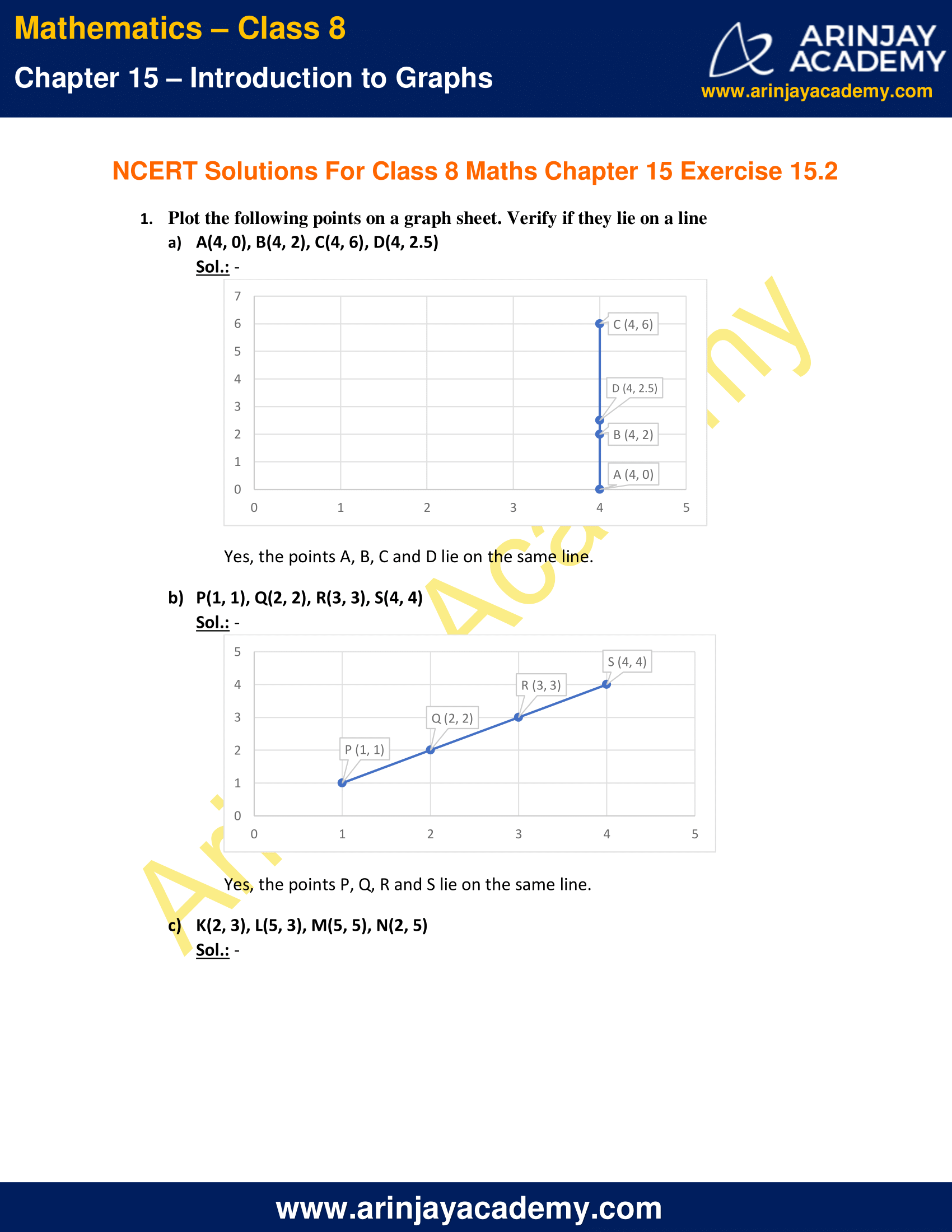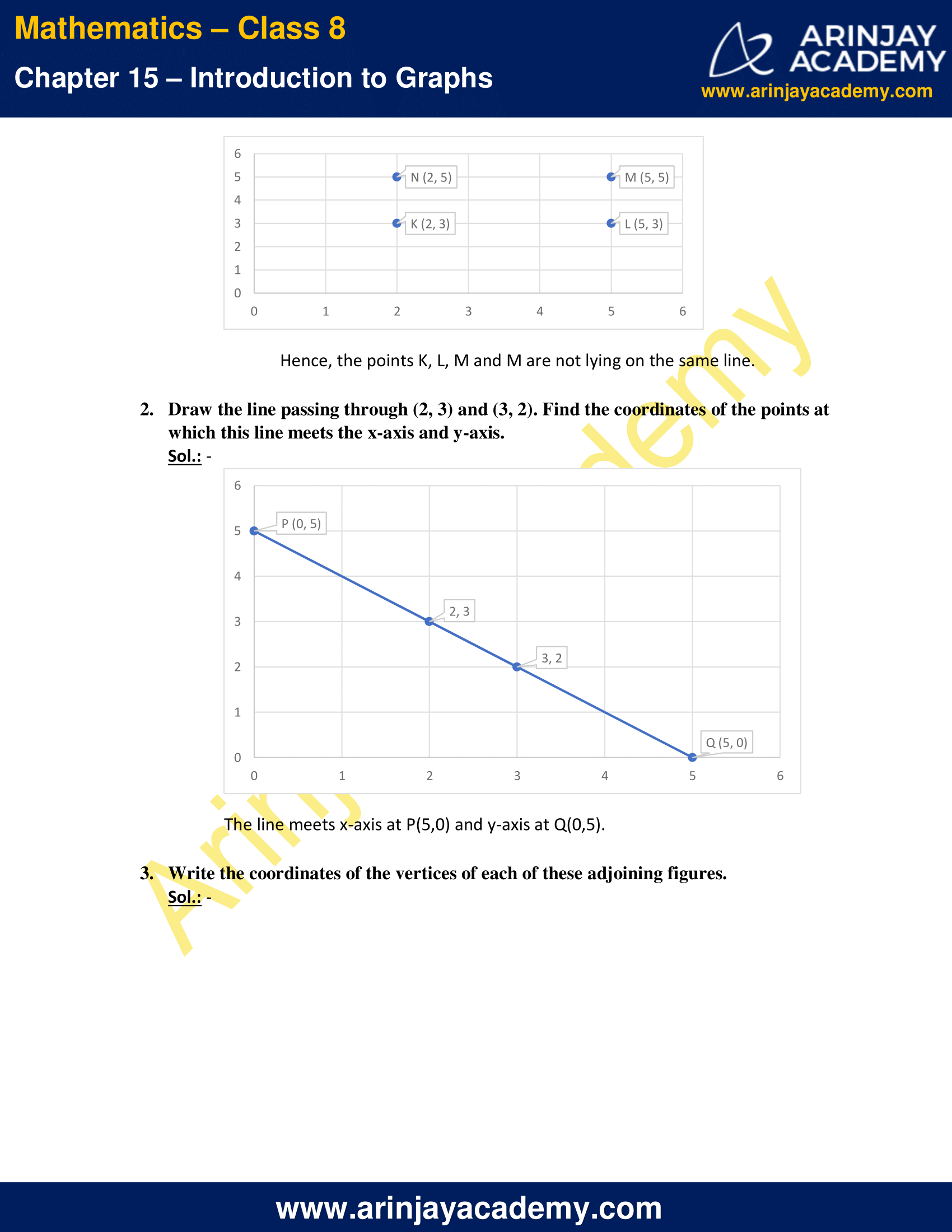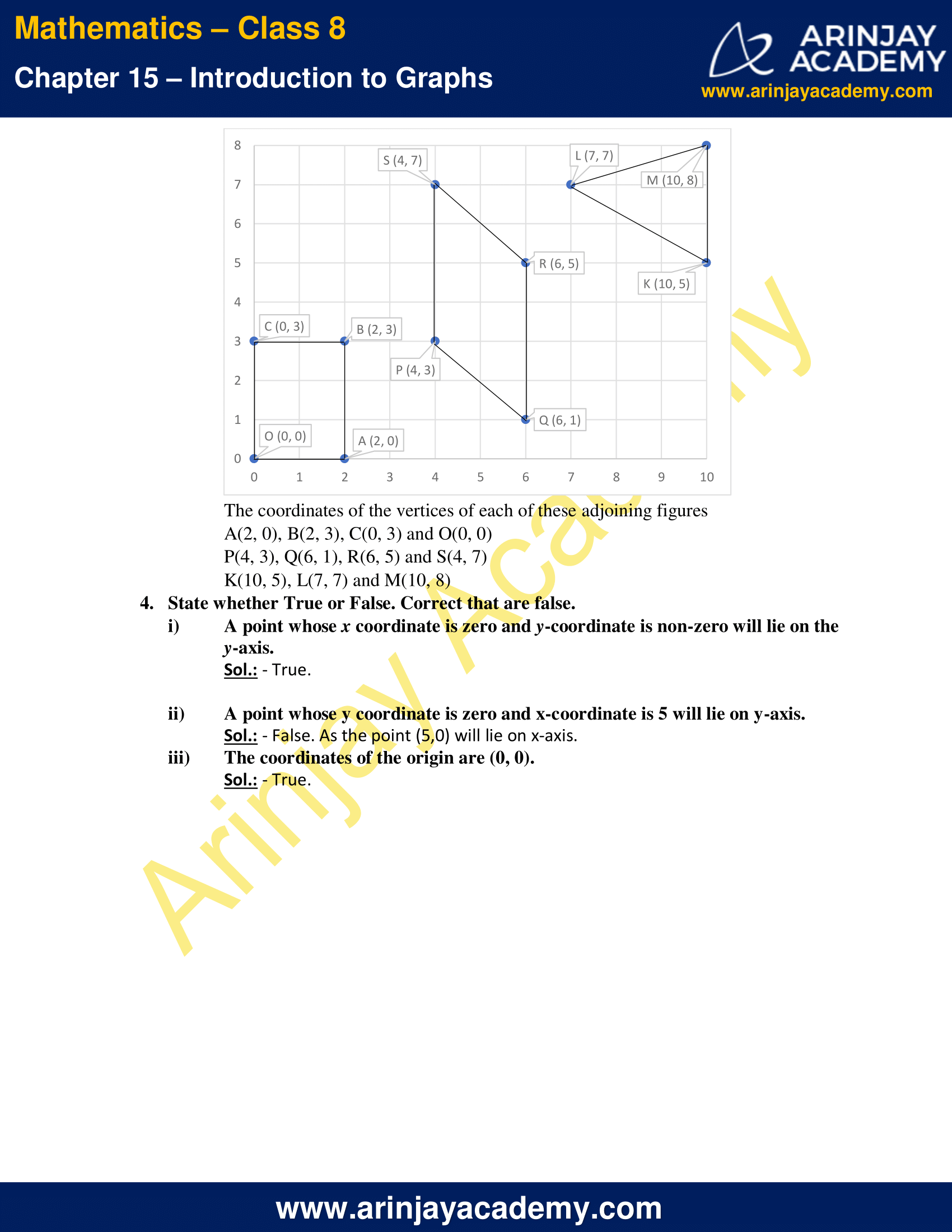NCERT Solutions for Class 8 Maths Chapter 15 Exercise 15.2 – Introduction to Graphs

1. Plot the following points on a graph sheet. Verify if they lie on a line

a) A(4, 0), B(4, 2), C(4, 6), D(4, 2.5)

Sol.: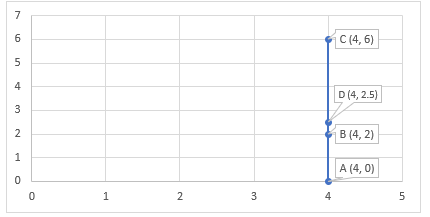Yes, the points A, B, C and D lie on the same line.

b) P(1, 1), Q(2, 2), R(3, 3), S(4, 4)

Sol.: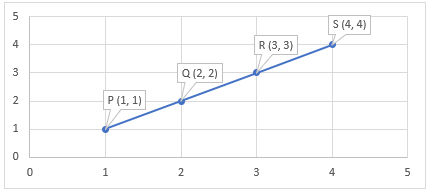Yes, the points P, Q, R and S lie on the same line.

c) K(2, 3), L(5, 3), M(5, 5), N(2, 5)

Sol.: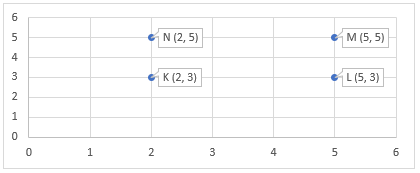Hence, the points K, L, M and M are not lying on the same line.

2. Draw the line passing through (2, 3) and (3, 2). Find the coordinates of the points at which this line meets the x-axis and y-axis.

Sol.: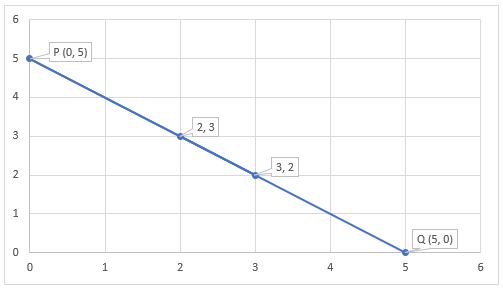The line meets x-axis at P(5,0) and y-axis at Q(0,5).

3. Write the coordinates of the vertices of each of these adjoining figures.

Sol.: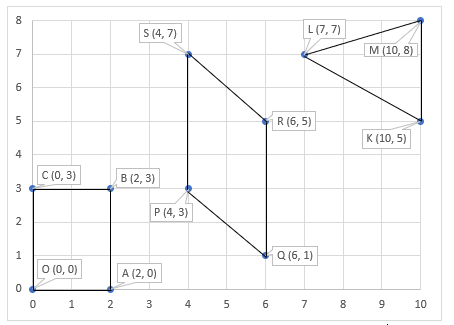The coordinates of the vertices of each of these adjoining figures
A(2, 0), B(2, 3), C(0, 3) and O(0, 0)
P(4, 3), Q(6, 1), R(6, 5) and S(4, 7)
K(10, 5), L(7, 7) and M(10, 8)

4. State whether True or False. Correct that are false.

i) A point whose x coordinate is zero and y-coordinate is non-zero will lie on the y-axis.
Sol.: – True.

ii) A point whose y coordinate is zero and x-coordinate is 5 will lie on y-axis.
Sol.: – False. As the point (5,0) will lie on x-axis.

iii) The coordinates of the origin are (0, 0).
Sol.: – True.

The next Exercise for NCERT Solutions for Class 8 Maths Chapter 15 Exercise 15.3 – Introduction to Graphs can be accessed by clicking here

Download NCERT Solutions for Class 8 Maths Chapter 15 Exercise 15.2 – Introduction to Graphs